# QLineF¶

The `QLineF` class provides a two-dimensional vector using floating point precision. More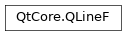## Detailed Description¶

A `QLineF` describes a finite length line (or line segment) on a two-dimensional surface. `QLineF` defines the start and end points of the line using floating point accuracy for coordinates. Use the `toLine()` function to retrieve an integer based copy of this line.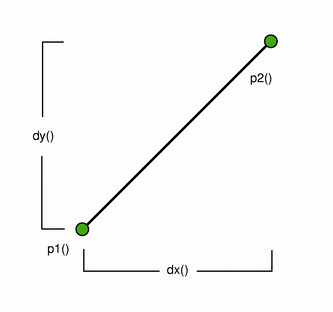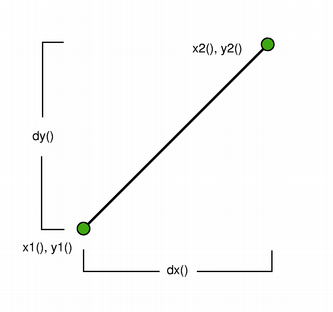The positions of the line’s start and end points can be retrieved using the `p1()` , `x1()` , `y1()` , `p2()` , `x2()` , and `y2()` functions. The `dx()` and `dy()` functions return the horizontal and vertical components of the line, respectively.

The line’s length can be retrieved using the `length()` function, and altered using the `setLength()` function. Similarly, `angle()` and `setAngle()` are respectively used for retrieving and altering the angle of the line. Use the `isNull()` function to determine whether the `QLineF` represents a valid line or a null line.

The `intersects()` function determines the IntersectionType for this line and a given line, while the `angleTo()` function returns the angle between the lines. In addition, the `unitVector()` function returns a line that has the same starting point as this line, but with a length of only 1, while the `normalVector()` function returns a line that is perpendicular to this line with the same starting point and length.

Finally, the line can be translated a given offset using the `translate()` function, and can be traversed using the `pointAt()` function.

### Constraints¶

`QLine` is limited to the minimum and maximum values for the `int` type. Operations on a `QLine` that could potentially result in values outside this range will result in undefined behavior.

`QLine` `QPolygonF` `QRectF`

class PySide2.QtCore.QLineF

PySide2.QtCore.QLineF(line)

PySide2.QtCore.QLineF(QLineF)

PySide2.QtCore.QLineF(pt1, pt2)

PySide2.QtCore.QLineF(x1, y1, x2, y2)

param QLineF:

`PySide2.QtCore.QLineF`

param y1:

float

param line:

`PySide2.QtCore.QLine`

param y2:

float

param pt1:

`PySide2.QtCore.QPointF`

param x1:

float

param pt2:

`PySide2.QtCore.QPointF`

param x2:

float

Constructs a null line.

Construct a `QLineF` object from the given integer-based `line` .

Constructs a line object that represents the line between `p1` and `p2` .

Constructs a line object that represents the line between (`x1` , `y1` ) and (`x2` , `y2` ).

PySide2.QtCore.QLineF.IntersectType

Constant

Description

QLineF.NoIntersection

Lines do not intersect.

QLineF.UnboundedIntersection

Lines intersect, but not within the range defined by their lengths.

QLineF.BoundedIntersection

Lnes intersect within the range defined by their lengths.

PySide2.QtCore.QLineF.__reduce__()
Return type:

object

PySide2.QtCore.QLineF.__repr__()
Return type:

object

PySide2.QtCore.QLineF.angle()
Return type:

float

Returns the angle of the line in degrees.

The return value will be in the range of values from 0.0 up to but not including 360.0. The angles are measured counter-clockwise from a point on the x-axis to the right of the origin (x > 0).

PySide2.QtCore.QLineF.angle(l)
Parameters:
Return type:

float

Note

This function is deprecated.

Returns the angle (in degrees) between this line and the given `line` , taking the direction of the lines into account. If the lines do not intersect within their range, it is the intersection point of the extended lines that serves as origin (see `UnboundedIntersection` ).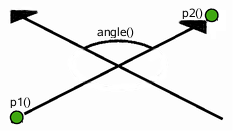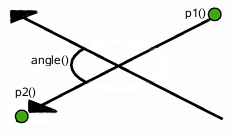When the lines are parallel, this function returns 0 if they have the same direction; otherwise it returns 180.

PySide2.QtCore.QLineF.angleTo(l)
Parameters:
Return type:

float

Returns the angle (in degrees) from this line to the given `line` , taking the direction of the lines into account. If the lines do not intersect within their range, it is the intersection point of the extended lines that serves as origin (see `UnboundedIntersection` ).

The returned value represents the number of degrees you need to add to this line to make it have the same angle as the given `line` , going counter-clockwise.

PySide2.QtCore.QLineF.center()
Return type:

`PySide2.QtCore.QPointF`

Returns the center point of this line. This is equivalent to 0.5 * `p1()` + 0.5 * `p2()` .

PySide2.QtCore.QLineF.dx()
Return type:

float

Returns the horizontal component of the line’s vector.

PySide2.QtCore.QLineF.dy()
Return type:

float

Returns the vertical component of the line’s vector.

static PySide2.QtCore.QLineF.fromPolar(length, angle)
Parameters:
• length – float

• angle – float

Return type:

`PySide2.QtCore.QLineF`

Returns a `QLineF` with the given `length` and `angle` .

The first point of the line will be on the origin.

Positive values for the angles mean counter-clockwise while negative values mean the clockwise direction. Zero degrees is at the 3 o’clock position.

PySide2.QtCore.QLineF.intersect(l)
Parameters:
Return type:

(intersectType, intersectionPoint)

Note

This function is deprecated.

Use `intersects()` instead

Returns a value indicating whether or not this line intersects with the given `line` .

The actual intersection point is extracted to `intersectionPoint` (if the pointer is valid). If the lines are parallel, the intersection point is undefined.

PySide2.QtCore.QLineF.intersects(l, intersectionPoint)
Parameters:
Return type:

`IntersectType`

Returns a value indicating whether or not this line intersects with the given `line` .

The actual intersection point is extracted to `intersectionPoint` (if the pointer is valid). If the lines are parallel, the intersection point is undefined.

PySide2.QtCore.QLineF.isNull()
Return type:

bool

Returns `true` if the line does not have distinct start and end points; otherwise returns `false` . The start and end points are considered distinct if `qFuzzyCompare()` can distinguish them in at least one coordinate.

Note

Due to the use of fuzzy comparison, may return `true` for lines whose `length()` is not zero.

`qFuzzyCompare()` `length()`

PySide2.QtCore.QLineF.length()
Return type:

float

Returns the length of the line.

PySide2.QtCore.QLineF.normalVector()
Return type:

`PySide2.QtCore.QLineF`

Returns a line that is perpendicular to this line with the same starting point and length.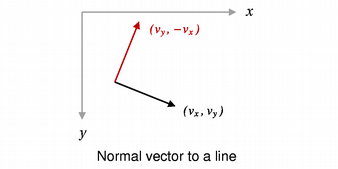PySide2.QtCore.QLineF.__ne__(d)
Parameters:
Return type:

bool

Returns `true` if the given `line` is not the same as this line.

A line is different from another line if their start or end points differ, or the internal order of the points is different.

PySide2.QtCore.QLineF.__mul__(m)
Parameters:
Return type:

`PySide2.QtCore.QLineF`

PySide2.QtCore.QLineF.__mul__(m)
Parameters:
Return type:

`PySide2.QtCore.QLineF`

PySide2.QtCore.QLineF.__eq__(d)
Parameters:
Return type:

bool

Returns `true` if the given `line` is the same as this line.

A line is identical to another line if the start and end points are identical, and the internal order of the points is the same.

PySide2.QtCore.QLineF.p1()
Return type:

`PySide2.QtCore.QPointF`

Returns the line’s start point.

PySide2.QtCore.QLineF.p2()
Return type:

`PySide2.QtCore.QPointF`

Returns the line’s end point.

PySide2.QtCore.QLineF.pointAt(t)
Parameters:

t – float

Return type:

`PySide2.QtCore.QPointF`

Returns the point at the parameterized position specified by `t` . The function returns the line’s start point if t = 0, and its end point if t = 1.

PySide2.QtCore.QLineF.setAngle(angle)
Parameters:

angle – float

Sets the angle of the line to the given `angle` (in degrees). This will change the position of the second point of the line such that the line has the given angle.

Positive values for the angles mean counter-clockwise while negative values mean the clockwise direction. Zero degrees is at the 3 o’clock position.

PySide2.QtCore.QLineF.setLength(len)
Parameters:

len – float

Sets the length of the line to the given `length` . `QLineF` will move the end point - `p2()` - of the line to give the line its new length, unless `length()` was previously zero, in which case no scaling is attempted. For lines with very short lengths (represented by denormal floating-point values), results may be imprecise.

PySide2.QtCore.QLineF.setLine(x1, y1, x2, y2)
Parameters:
• x1 – float

• y1 – float

• x2 – float

• y2 – float

Sets this line to the start in `x1` , `y1` and end in `x2` , `y2` .

PySide2.QtCore.QLineF.setP1(p1)
Parameters:

Sets the starting point of this line to `p1` .

PySide2.QtCore.QLineF.setP2(p2)
Parameters:

Sets the end point of this line to `p2` .

PySide2.QtCore.QLineF.setPoints(p1, p2)
Parameters:

Sets the start point of this line to `p1` and the end point of this line to `p2` .

PySide2.QtCore.QLineF.toLine()
Return type:

`PySide2.QtCore.QLine`

Returns an integer based copy of this line.

Note that the returned line’s start and end points are rounded to the nearest integer.

`QLineF()`

PySide2.QtCore.QLineF.toTuple()
Return type:

object

PySide2.QtCore.QLineF.translate(p)
Parameters:

Translates this line by the given `offset` .

PySide2.QtCore.QLineF.translate(dx, dy)
Parameters:
• dx – float

• dy – float

Translates this line the distance specified by `dx` and `dy` .

PySide2.QtCore.QLineF.translated(p)
Parameters:
Return type:

`PySide2.QtCore.QLineF`

Returns this line translated by the given `offset` .

PySide2.QtCore.QLineF.translated(dx, dy)
Parameters:
• dx – float

• dy – float

Return type:

`PySide2.QtCore.QLineF`

Returns this line translated the distance specified by `dx` and `dy` .

PySide2.QtCore.QLineF.unitVector()
Return type:

`PySide2.QtCore.QLineF`

Returns the unit vector for this line, i.e a line starting at the same point as this line with a length of 1.0, provided the line is non-null.

PySide2.QtCore.QLineF.x1()
Return type:

float

Returns the x-coordinate of the line’s start point.

PySide2.QtCore.QLineF.x2()
Return type:

float

Returns the x-coordinate of the line’s end point.

PySide2.QtCore.QLineF.y1()
Return type:

float

Returns the y-coordinate of the line’s start point.

PySide2.QtCore.QLineF.y2()
Return type:

float

Returns the y-coordinate of the line’s end point.